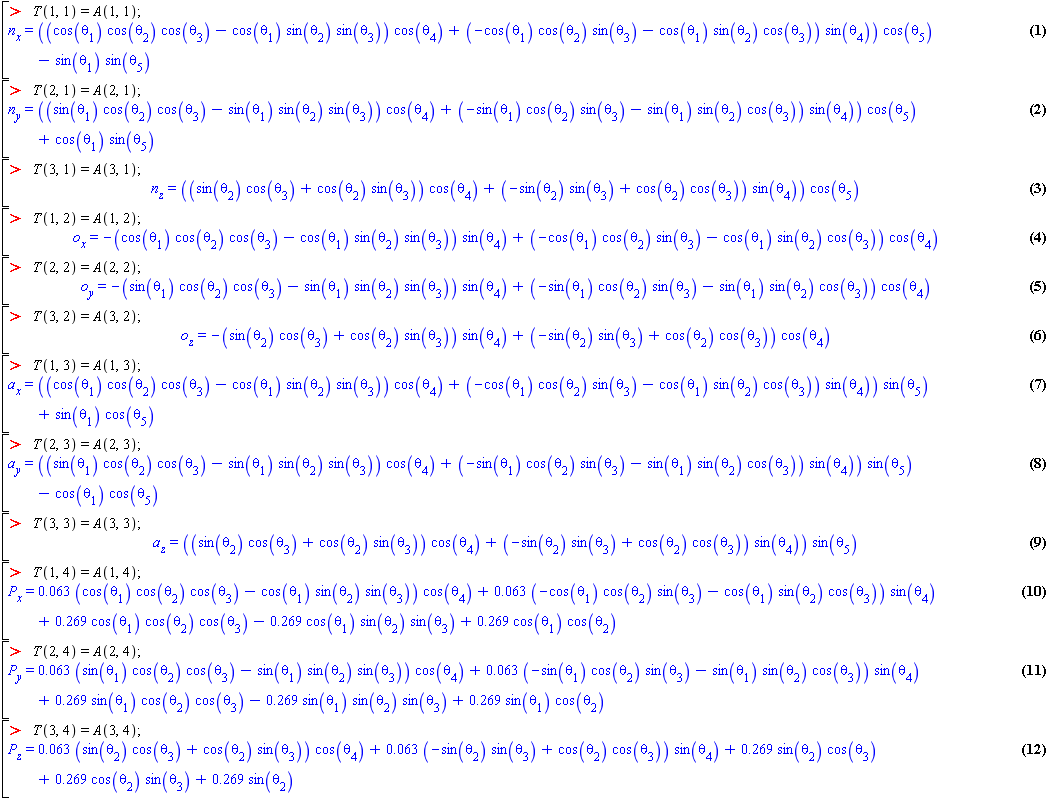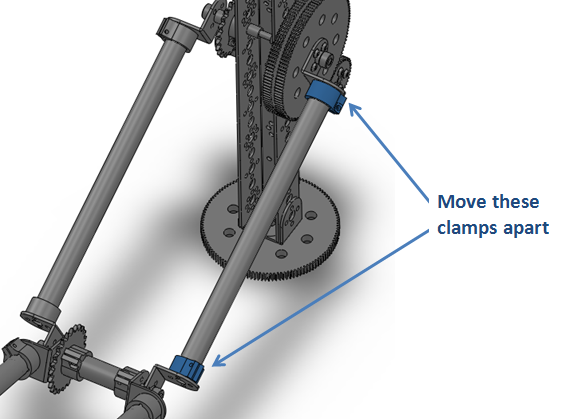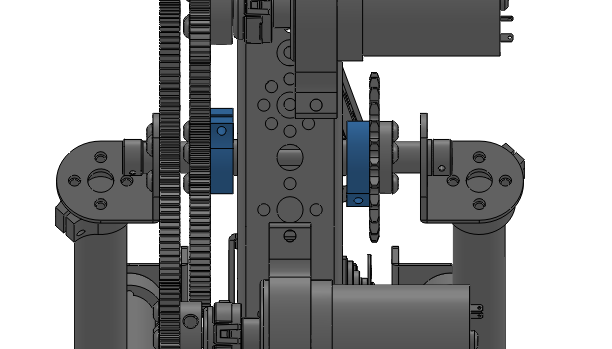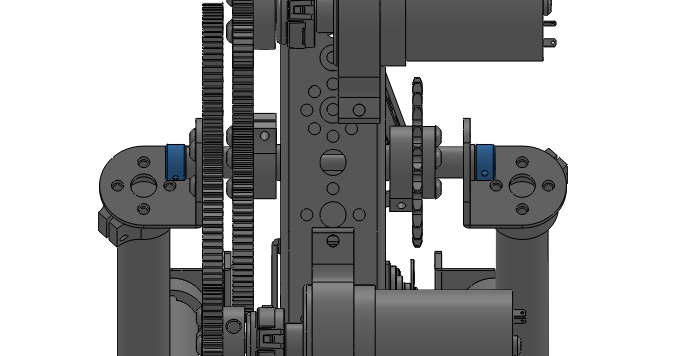# Combination Report Einstein Robot

 9 Arm Appendix References: Niku, A.B. (2001). Introduction to Robotics: Analysis, Systems, Applications. Upper Saddle River, New Jersey: Prentice Hall. Braunl, T. (2008). Embedded Robotics: Mobile Robot Design and Application with Embedded Systems. Berlin Heidelberg: Springer-Verlag. Hartenberg, Richard and Jacques Danavit.(1964). Kinematic Synthesis of Linkages New York: McGraw-Hill, 1964 Useful Links and Downloads: TETRIX solid model library (SolidWorks 2009): http://web.cecs.pdx.edu/~furnissj/tetrix_models.zip Source for TETRIX solid models: http://www.3dcontentcentral.com/Search.aspx?arg=tetrix Solid model of robot (SolidWorks 2009): http://web.cecs.pdx.edu/~furnissj/final_assembly.zip Optical encoder manufacture’s link: http://usdigital.com/products/encoders/incremental/rotary/kit/e4p/ Optical encoder data sheet: http://usdigital.com/products/encoders/incremental/rotary/kit/e4p/ TETRIX gear motor data sheet: http://web.cecs.pdx.edu/~furnissj/Tetrix%20DC%20drive%20motor.pdf C code: http://web.cecs.pdx.edu/~furnissj/armjoystick.c Photo gallery: http://www.flickr.com/photos/furnissj/sets/72157622850565385/ Video of final demo: http://web.cecs.pdx.edu/~furnissj/robot_arm.wmv RobotC code: #pragma config(Hubs, S1, HTMotor, HTServo, none, none) #pragma config(Motor, mtr_S1_C1_1, motorD, tmotorNormal, openLoop) #pragma config(Motor, mtr_S1_C1_2, motorE, tmotorNormal, openLoop) #pragma config(Servo, srvo_S1_C1_1, , tServoNormal) //*!!Code automatically generated by 'ROBOTC' configuration wizard !!*// /************************************************************************/ /* */ /* Program Name: PSP-Nx-motor-control.c */ /* =========================== */ /* */ /* Copyright (c) 2008 by mindsensors.com */ /* Email: info () mindsensors () com */ /* */ /* This program is free software. You can redistribute it and/or modify */ /* it under the terms of the GNU General Public License as published by */ /* the Free Software Foundation; version 3 of the License. */ /* Read the license at: http://www.gnu.org/licenses/gpl.txt */ /* */ /************************************************************************/ const ubyte Addr = 0x02; const tSensors SensorPort = S2; // Connect PSPNX sensor to this port!! #include "PSP-Nx-lib.c" int nLeftButton = 0; int nRightButton = 0; int nEnterButton = 0; int nExitButton = 0; int tri, squ, cir, cro, a, b, c; ////////////////////////////////////////////////////////////////////////////// // // Gaskin 11/1/09 Modified to work wth ECE578 program // ///////////////////////////////////////////////////////////////////////////// task main () { int powerD = 0; int powerE = 0; psp currState; // // Note: program cannot be terminated if we hijack the 'exit' button. So there has to be an escape sequence // that will return buttons to system control! We'll use a triple click // nNxtExitClicks = 3; // Triple clicking EXIT button will terminate program // nI2CBytesReady[SensorPort] = 0; //SensorType[SensorPort] = sensorI2CCustom9V; // or perhaps use 'Lowspeed_9V_Custom0'?? SensorType[SensorPort] = sensorI2CMuxController; wait10Msec (100); while ( true ) { wait1Msec (5); //Move shoulder up if ((int)currState.triang==0) { powerD = -50; tri=(int)currState.triang; } //Move shoulder down if ((int)currState.square==0) { powerD = 10; squ=(int)currState.square; } //Move elbow up if ((int)currState.circle==0) { powerE = -50; cir=(int)currState.circle; } //Move elbow down if ((int)currState.cross==0) { powerE = 5; cro=(int)currState.cross; } //Turn off motors if ((int)currState.cross==1 && (int)currState.circle==1 && (int)currState.square==1 && (int)currState.triang==1) { powerD = 0; powerE = 0; } //Move wrist L/R if ((int)currState.r_j_y<-50) { servo[servo1]=ServoValue[servo1]+2; while(ServoValue[servo1] != servo[servo1]) { a=ServoValue[servo1]; } } if ((int)currState.r_j_y>50) { servo[servo1]=ServoValue[servo1]-2; while(ServoValue[servo1] != servo[servo1]) { a=ServoValue[servo1]; } } //Move wrist U/D if ((int)currState.r_j_x<-50) { servo[servo2]=ServoValue[servo2]-2; while(ServoValue[servo2] != servo[servo2]) { b=ServoValue[servo2]; } } if ((int)currState.r_j_x>50) { servo[servo2]=ServoValue[servo2]+2; while(ServoValue[servo2] != servo[servo2]) { b=ServoValue[servo2]; } } //Close hand if ((int)currState.r1==0) { servo[servo3]=ServoValue[servo3]+2; while(ServoValue[servo3] != servo[servo3]) { c=ServoValue[servo3]; } } //Open hand if ((int)currState.l1==0) { servo[servo3]=ServoValue[servo3]-2; while(ServoValue[servo3] != servo[servo3]) { c=ServoValue[servo3]; } } //Move arm right if ((int)currState.r2==0) { servo[servo4]=ServoValue[servo4]+2; while(ServoValue[servo4] != servo[servo4]) { d=ServoValue[servo4]; } } //Move arm left if ((int)currState.l2==0) { servo[servo4]=ServoValue[servo4]-2; while(ServoValue[servo4] != servo[servo4]) { d=ServoValue[servo4]; } } } wait10Msec (100); StopAllTasks (); } MATLAB code: function position_orientation = forward_kinematics(joint_angles) % =================================================== % forward_kinematics calculates the pos and orientation of the end-effector for a 5 DOF open kinematic chain. % % Input: joint_angles = [theta1 theta2 theta3 theta5 theta5] % 1x5 vector of joint angles measured in degrees % % Output: position_orientation = [nx ny nz ox oy oz ax ay az Px Py Pz] % 1x12 vector containing components of unit vectors, (a,n,o) % that define the x,y, and z axies of the end-effector (orientation) % and componented of a vector P that defines the position % =================================================== joint_angles = (pi/180).*joint_angles; % convert to radians theta1 = joint_angles(1); theta2 = joint_angles(2); theta3 = joint_angles(3); theta4 = joint_angles(4); theta5 = joint_angles(5); % link lengths [meters]: a1 = 0; a2 = 0.269; a3 = 0.269; a4 = 0.063; a5 = 0; % joint offsets [meters]: d1 = 0; d2 = 0; d3 = 0; d4 = 0; d5 = 0; % angles between sucessive joints [radians]: alpha1 = pi/2; alpha2 = 0; alpha3 = 0; alpha4 = -pi/2; alpha5 = pi/2; % transformation matricies between sucessive frames: A1 = [cos(theta1) -sin(theta1)*cos(alpha1) sin(theta1)*sin(alpha1) a1*cos(theta1); sin(theta1) cos(theta1)*cos(alpha1) -cos(theta1)*sin(alpha1) a1*sin(theta1); 0 sin(alpha1) cos(alpha1) d1 ; 0 0 0 1] ; A2 = [cos(theta2) -sin(theta2)*cos(alpha2) sin(theta2)*sin(alpha2) a2*cos(theta2); sin(theta2) cos(theta2)*cos(alpha2) -cos(theta2)*sin(alpha2) a2*sin(theta2); 0 sin(alpha2) cos(alpha2) d2 ; 0 0 0 1] ; A3 = [cos(theta3) -sin(theta3)*cos(alpha3) sin(theta3)*sin(alpha3) a3*cos(theta3); sin(theta3) cos(theta3)*cos(alpha3) -cos(theta3)*sin(alpha3) a3*sin(theta3); 0 sin(alpha3) cos(alpha3) d3 ; 0 0 0 1] ; A4 = [cos(theta4) -sin(theta4)*cos(alpha4) sin(theta4)*sin(alpha4) a4*cos(theta4); sin(theta4) cos(theta4)*cos(alpha4) -cos(theta4)*sin(alpha4) a4*sin(theta4); 0 sin(alpha4) cos(alpha4) d4 ; 0 0 0 1] ; A5 = [cos(theta5) -sin(theta5)*cos(alpha5) sin(theta5)*sin(alpha5) a5*cos(theta5); sin(theta5) cos(theta5)*cos(alpha5) -cos(theta5)*sin(alpha5) a5*sin(theta5); 0 sin(alpha5) cos(alpha5) d5 ; 0 0 0 1] ; % total transformation matrix: A = A1*A2*A3*A4*A5; A = A(1:3,:); %eliminate bottom row position_orientation = reshape(A,1,[]); % [nx ny nz ox oy oz ax ay az Px Py Pz]] % round down small numbers tolerance = 0.0001; for i = 1:length(position_orientation) if abs(position_orientation(i)) < tolerance position_orientation(i) = 0; end end Forward Kinematic Equations:Trouble Shooting Guide Although we didn’t experience any major technical difficulties after the arm was built and tested, there are a few aspects of the assembly that may need attention after more use. The overall structure of the arm is pushing the bounds of the TETRIX components. To keep the arm in good working condition the following should be considered. At full extension, the motors have to resist a torque that is very near their limit. For this reason, motions that are programmed into the arm must be very slow. Quick movements (accelerations) increase the risk of stripping, bending, or loosening components. If you want the arm to move faster or carry a greater load, a first step would be to make it smaller by shortening the tubes. The chain is tensioned by positioning the tube clamps at the ends of the tubes on the upper arm. When there is load on the arm, the chain will become loose due to flex in the system. This can’t be avoided. If the chain becomes excessively loose, follow these steps to adjust it. Loosen the set screw that binds the 40T gear to the DC motor shaft that controls the elbow joint. This will make the elbow joint move freely. Loosen the 4 tubing clamps on the upper arm. Make sure it doesn’t fall apart. On the non-chain side, slide the clamps away from the center of the tube a couple of millimeters and re-tighten the clamps. See below. The tubing plugs may slide away from the ends of the tube when you loosen the clamps. Make sure you slide them back before you retighten the clamps.Do the same on the other side. As you move the clamps away, you will feel the chain getting tight. Make the chain as tight as you can without sliding the clamp off the end of the tube. This process it a bit awkward and may take several attempts. Make sure you don’t rotate the clamps relative to one another. This will put a twist in the arm. Re-tighten the set screw on the 40T gear. Make sure the screw is against the flat on the motor shaft. The main shoulder pivot may become loose. Follow these steps to correct the problem. Loosen the set screws that bind the inside split clamps to the main axle. These are highlighted in the figure below. On the real robot, these pieces have been modifies and don’t look exactly the same as in the figure.Sandwich these components together and simultaneously tighten the set screws. This will take two people. Try to get at least one setscrew on each clamp to tighten against the flat on the axle. Now, do the same thing with the axle set collars. See figure below.My final word of advice is in regard to the supposed position control of the DC motors with RobotC. There is no PID algorithm for position control, only for speed. After reading everything I could find about this on RobotC forums and in the documentation, there does not seem to be a reliable way to command the motors to a position. The main approach that I found was to drive the motor at a constant speed (with the PID speed control) in a while loop until some encoder value was reached. If you want to implement some sophisticated method of motion control with the robot, I don’t think this will cut it. If you read about other’s frustrations with this on the forums, you will see that it hasn’t cut it for anyone else ether. My advice would be to design a controller on your own unless you find successful solution on the internet. Share with your friends: